## Tuesday, February 28, 2012

### Determining if the basal node of a tree leaves descendants from both its right & left daughters

A colleague contacted me last night to ask the following:

"Can you think of a quick way to test whether, in a simulated birth-death tree, both lineages descending from the basal node are represented in the extant taxa?"

In other words, can we distinguish:

> require(geiger)
> tr1<-birthdeath.tree(b=1,d=0.3,taxa.stop=20)
> plotTree(tr1,ftype="off",pts=F)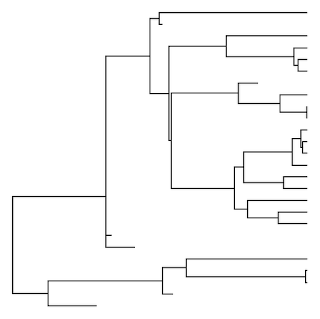from:

> tr2<-birthdeath.tree(b=1,d=0.3,taxa.stop=20)
> plotTree(tr2,ftype="off",pts=F)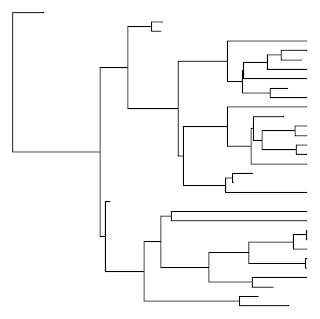(aside from, say, by visual inspection)?

It immediately occurred to me that asking if both the right and left daughters of the root node have left descendants among the extant taxa is the same as asking if the MRCA of all the extant taxa in the tree was equal to the root node. By chance, in response to another user request I recently wrote a different function, findMRCA, that returns the MRCA for a list of species. All, then, that was needed was a function to pull out a list of the extant taxa in the tree. There is a couple of different ways I could envision doing this, but the following is what I settled on:

getExtant<-function(tree,tol=1e-8){
H<-nodeHeights(tree)
tl<-max(H)
x<-which(H[,2]>=(tl-tol))
y<-tree\$edge[x,2]
y<-y[y<=length(tree\$tip)]
z<-tree\$tip.label[y]
return(z)
}
getExtinct<-function(tree,tol=1e-8)
setdiff(tree\$tip.label,getExtant(tree,tol))

The way this function works is by first using nodeHeights to get the height above the root of all the internal and terminal nodes in the tree. It then gets the node numbers of the extant taxa by finding all the terminal nodes that are (within a certain tolerance) equal in height to the maximum height of the tree. These node numbers are then used to pull the tip labels of all the extant taxa from tree\$tip.label.

Having loaded the above function into memory, we can then ask if the node number of the root (which is always equal to length(tree\$tip)+1) is equal to the MRCA of the extant taxa in the tree as follows:

> require(phytools) # phytools 0.1-7 or above
> (length(tr1\$tip)+1)==findMRCA(tr1,getExtant(tr1)) # expect TRUE
 TRUE
> (length(tr2\$tip)+1)==findMRCA(tr2,getExtant(tr2)) # expect FALSE
 FALSE

Cool.

## Saturday, February 25, 2012

### New version of phytools submitted to CRAN

I just submitted a new version of phytools (v0.1-7) to CRAN. Assuming this version is approved, it will most likely percolate onto the CRAN mirrors within a few days.

New features relative to the last CRAN version of phytools include the following (in no particular order):

1) A new function, findMRCA, which uses the ape function mrca and phytools function nodeHeights to find the set of MRCAs for a list of tips. If findMRCA(tree,tips=NULL) (the default) then the function just returns mrca(tree). My blog post about this function is here.

2) An update to plotSimmap in which the underscore character, "_" is swapped for a blank space (i.e., " ") in tip labels for plotting. Note, this will also affect plotTree. I wrote about how this could be done externally to the function here, and Dave Bapst suggested I add this to the function - and now I have.

3) A new function called paintSubTree that is described here and here.

4) A new version of phylosig in which control of multivariate optimization for phylosig(...,method="lambda",se!=NULL) is migrated to the user. I also made some attempt to improve the default starting values for optimization under this model. These changes are described here (with the bug report that inspired it here).

5) A new version of phyl.RMA for phylogenetic major axis regression which also includes hypothesis testing about the regression slope. This is described here. This change was requested by a phytools user.

6) A new version of phenogram which allows the user to control the axis dimensions. This update was motivated by a user request and is described here.

7) A major update to brownie.lite which allows the incorporation of information about intraspecific sampling error following Ives et al. (2007). This involved a total re-write of the function. The new version is described here. The function is, of course, based on the method by O'Meara et al. (2006).

9) An update to plotSimmap which allows the user to plot node numbers for internal branching points in the tree. This is described here.

10) A minor change to plotTree, now really just a wrapper for plotSimmap, which allows it to inherit the ability of plotSimmap to plot node numbers.

I think that's about it. Hopefully the new version of phytools will be available from CRAN shortly. (Check the phytools CRAN page.) In the meantime, interested users can also download the package source here and install as follows:

> install.packages("phytools_0.1-7.tar.gz",type="source", repos=NULL)

Thanks for trying out phytools and reporting bugs!

## Thursday, February 23, 2012

### MRCA for a set of taxa

Do you know any function that could allow finding the deepest node ancestral to a group of tips? (I mean the oldest MRCA of a group of tips.)

The ape function mrca computes the pairwise most recent common ancestors for all the tips in a tree; however how do we take a set of pairwise MRCAs and identify the one that is also the MRCA of the whole group?

Well, by convention in "phylo" objects, node numbers increase along any path from the node. Logically, therefore, we ought to be able to pick the pairwise MRCA with the lowest number as the MRCA of a list. Since "phylo" objects are somewhat flexible, I decided to be careful and construct a function that pairs the ape function mrca with the phytools function nodeHeights to find the node in a set of pairwise MRCAs that is closest to the root node (i.e., it has the lowest height). Also, logically, this should be the MRCA of a set of tips.

The function looks as follows:

oldest.mrca<-function(tree,tips){
H<-nodeHeights(tree)
X<-mrca(tree)
n<-length(tips)
nodes<-height<-vector(); k<-1
for(i in 1:(n-1)) for(j in (i+1):n){
nodes[k]<-X[tips[i],tips[j]]
height[k]<-H[match(nodes[k],tree\$edge[,1]),1]
k<-k+1
}
z<-match(min(height),height)
return(nodes[z])
}

Let's load the function & phytools and then see how it works. First on an ultrametric tree (simulated using phytools pbtree):

> tree<-pbtree(n=20)
> plotTree(tree,node.numbers=T,pts=F)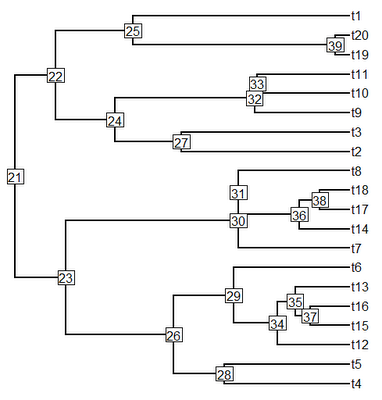> oldest.mrca(tree,c("t14","t18")) # two taxa case
 36
> oldest.mrca(tree,c("t1","t3","t19"))
 22
> oldest.mrca(tree,c("t2","t3","t9","t11"))
 24
> oldest.mrca(tree,c("t2","t3","t4"))
 21

Now, let's try the same with a non-ultrametric tree:

> tree<-rtree(20)
> plotTree(tree,node.numbers=T,pts=F)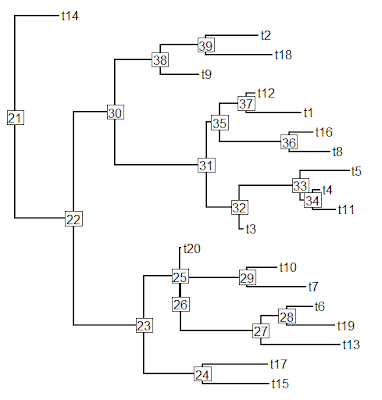> oldest.mrca(tree,c("t2","t12"))
 30
> oldest.mrca(tree,c("t14","t19","t20"))
 21
> oldest.mrca(tree,c("t10","t13","t20"))
 25

Seems to work. . . .

## Tuesday, February 21, 2012

### Michael Nielsen now speaking on NPR about "open science"

Just as I was (at least to some degree) partaking in "open science" - that is, blogging on phytools-blog - I noticed that Michael Nielsen is now speaking on NPR's "On Point" program about open science. See an article about this interview here. If you happen to read this post in time, you can listen-in here.

### Adding spaces between genus & specific names in tree objects

Newick format typically excludes spaces within species & node labels on the tree; however we would often like to plot binomial species names with a space between them. This is done by default in ape's plot.phylo if the "_" character is used in lieu of a space. For instance:

> plot.phylo(tree)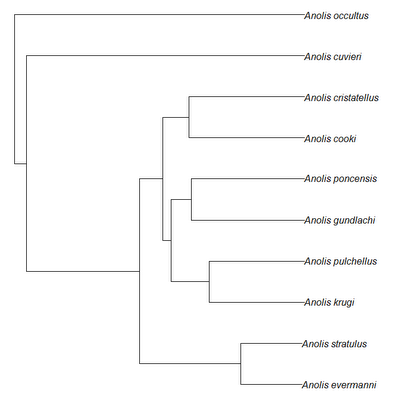This does not work with phytools functions plotTree and plotSimmap, in which the whole string is written. However, there is no constraint on "phylo" objects in R requiring that tip labels lack spaces, so we can add them if we want before plotting.

> tree\$tip.label<-gsub("_"," ",tree\$tip.label)
> plotTree(tree,node.numbers=T,pts=F,ftype="i")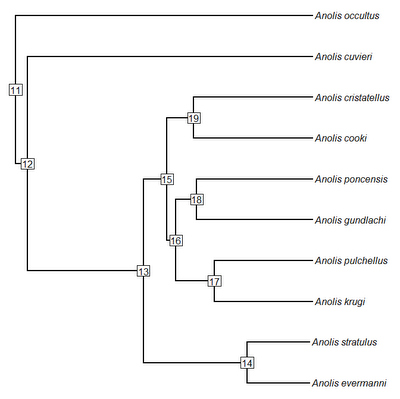For fun, let's paint the Puerto Rican Anolis ecomorphs on the tree - arbitrarily assigning the stem branches to the state of the descendant node, and the root state to "TG" (i.e., the trunk-ground ecomorph):

> tree<-paintSubTree(tree,11,"TG")
> tree<-paintSubTree(tree,which(tree\$tip.label=="Anolis occultus"), "Tw",stem=T)
> tree<-paintSubTree(tree,which(tree\$tip.label=="Anolis cuvieri"), "CG",stem=T)
> tree<-paintSubTree(tree,which(tree\$tip.label=="Anolis poncensis"), "GB",stem=T)
> tree<-paintSubTree(tree,17,"GB",stem=T)
> tree<-paintSubTree(tree,14,"TC",stem=T)
> eco<-c("TG","Tw","CG","GB","TC")
> cols<-c("red","grey","green","yellow","blue")
> names(cols)<-eco
> plotSimmap(tree,cols,pts=F,lwd=4,ftype="i")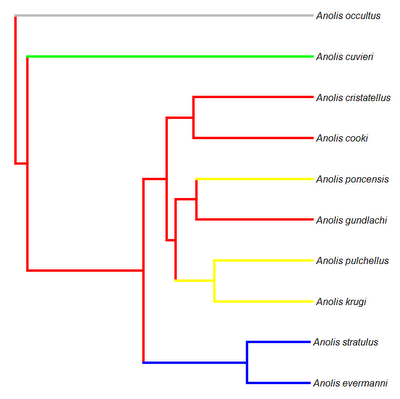Cool.

## Wednesday, February 15, 2012

### Likelihood methods for comparative biology incorporating uncertainty in the estimation of species means

A recent search string that lead a user to this blog was as follows:

"comparative phylogenetics standard error or standard deviation"

By this, I presume they were trying to figure out whether functions such as fitContinuous in the geiger package; or phylosig and brownie.lite in phytools (in which we are fitting an evolutionary model for the evolution of the phenotypic mean of a continuously valued trait to the data for species means and the tree) accepts measures of uncertainty for species as the standard errors of the species means or the standard deviations of the observations for each species. Obviously, the parametric estimate of the former is just the latter divided by the square-root of the sample size for each species.

The answer is that we use the standard errors of the means for each species in these analyses, not the standard deviations. It is only the former that tells us how uncertain we are about our estimates of the means for species. This is because even for very large standard deviation σ, our uncertainty about any given sample mean goes to zero as n → ∞. Parametric estimates of σ, by contrast, are theoretically unaffected by sample size.

I hope that the previous searcher found what he or she was looking for, and now it should be even easier!

## Monday, February 13, 2012

### New version of phytools

I just posted a new version of the phytools online. I plan to add a couple of more things, including vignettes, before submitting the next CRAN version of the package, but if you would like to get a new version of phytools sooner - please download the source version from the following link. You can then install by navigating to the directory containing the package source file and then typing:

> install.packages("phytools_0.1-64.tar.gz",type="source", repos=NULL)

## Sunday, February 12, 2012

### New version of paintSubTree

I just posted a new version of the function paintSubTree which can be used to arbitrarily paint different parts of the tree in the same format as my other functions that map discrete characters on the phylogeny (e.g., read.simmap, make.simmap, and sim.history). The reason I developed this function was mainly so that users could map arbitrary rate regimes (for instance, a specific taxonomic clade) and then use this mapping in functions such as brownie.lite and evol.vcv, but it is also handy for coloring parts of the phylogeny and then plotting these using plotSimmap.

The updated version of this function does a couple of things.

First, it is no longer necessary to provide a tree with branch lengths. Instead, if desired, users can supply a tree without branch lengths and branch lengths will be computed using the ape function compute.brlen. This function (by default) assigns the same branch lengths that one would see if visually a tree without branch lengths using plot.phylo in the ape package.

Second, this version of the function can color parts (instead of just the whole) of the stem branch for the painted sub-tree. Since TRUE and FALSE are treated as 1 & 0 during calculations in R, this change is totally backward compatible with the previous version of paintSubTree in which a logical value for the argument stem was provided.

Finally, third, the function fixes a bug that was identified by Sebastien Lavergne that came up whenever we tried to map one regime on top of a branch upon which the regime had changed. This was a book-keeping error that it was necessary to fix anyway in the process of adding the functionality of the second item, above.

Direct link to the code for the new version is here.

Let's try out this function:

> require(phytools)
> source("paintSubTree.R")
> tree<-pbtree(n=10)
> plotTree(tree,node.numbers=T,pts=F)> tree<-paintSubTree(tree,node=15,stem=0.8,state=2)
> plotSimmap(tree,node.numbers=T,pts=F,lwd=3)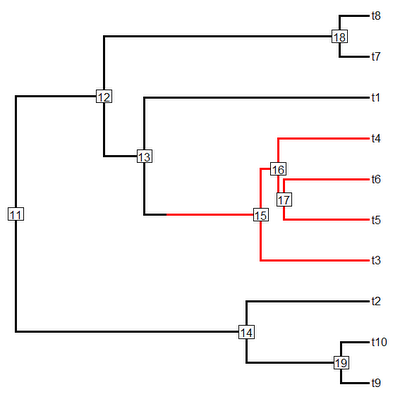> tree<-paintSubTree(tree,node=15,stem=0.2,state=3)
> plotSimmap(tree,node.numbers=T,pts=F,lwd=3)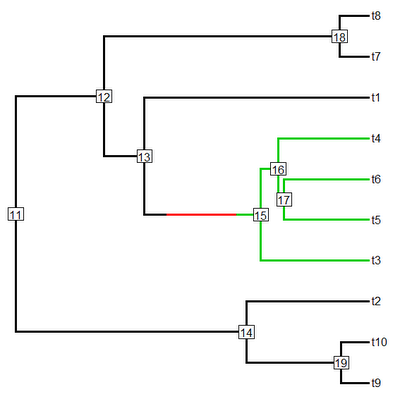> tree<-paintSubTree(tree,node=18,stem=T,state=2)
> plotSimmap(tree,node.numbers=T,pts=F,lwd=3)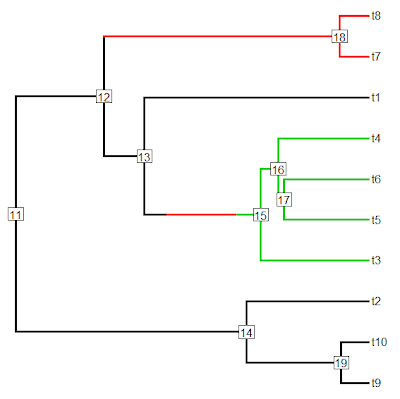> tree<-paintSubTree(tree,node=match("t6",tree\$tip.label), stem=0.8,state=2)
> plotSimmap(tree,node.numbers=T,pts=F,lwd=3)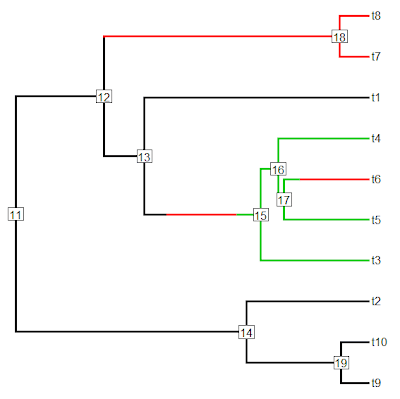Cool. Please let me know if you find any more bugs!

## Saturday, February 11, 2012

### Quicker way to rescale the total length of a tree

In my function pbtree I provide the option to rescale the total length (i.e., height) of the tree to an arbitrary value. This is also available in the geiger function rescaleTree. The only problem with this latter option is that rescaleTree works by dividing tree\$edge.length by the maximum value in the diagonal of vcv.phylo(tree). Unfortunately the calculation of vcv.phylo(tree) is not too fast for large trees.

In phytools there is a function nodeHeights which computes in a matrix of dimensions dim(tree\$edge) the corresponding heights above the root for all the nodes in tree\$edge. That means that to rescale the tree to have height scale we can just do:

tree\$edge.length<-
tree\$edge.length/max(nodeHeights(tree)[,2])*scale

This is indeed much faster than rescaleTree.

For instance, on my VAIO laptop:

> require(phytools); require(geiger)
> tree<-pbtree(n=2000)
> # rescale tree to length 100
> system.time(tr1<-rescaleTree(tree,100))
user  system elapsed
118.43    0.14  123.15
> # now let's submit an alternative version
> rescaleTree<-function(tree,scale){
tree\$edge.length<-
tree\$edge.length/max(nodeHeights(tree)[,2])*scale
return(tree)
}
> system.time(tr2<-rescaleTree(tree,100))
user  system elapsed
2.49    0.06    2.56
> all.equal.phylo(tr1,tr2)
 TRUE

That's it.

### New version of phylosig

I just posted a new version of phylosig with a couple of minor updates.

First there was a bug (due to a misspelling) that caused the function to return NULL for convergence, even if it had in fact converged.

Second, a user pointed out that I had not specified what good starting values for the optimization might be. Let me say that I have tried to improve the default starting values for optimization in the phylosig(...,method="lambda",se=SE) model, and specifying start is optionally. However, if you do want to specify start, it can be provided as a vector length 2 containing the starting values for optimization for σ2 and λ, respectively. Thus, the first value needs to be on the valid range for σ2 - that is, greater than 0. The second value should be between 0 & the maximum theoretical value of λ (this is usually very close to 1). Note that this will only used in the models that use optim, which is basically the λ with sampling error model.

Direct link to the code for this new version is here. I'm also planning to build a new version of phytools soon.

## Friday, February 10, 2012

### New version of phyl.RMA for reduced major axis regression

I just posted a new version of phyl.RMA which also computes r2, T, df, and a P value for the test. The r2 is just the squared phylogenetic correlation. The other values are based on McArdle (1987). Direct link to code is here.

By default, the function tests the null hypothesis that the RMA slope is equal to 1.0. It is possible to change this default; however it is generally not possible to test for β=0.0 as this would only be true if σY2 was zero.

Here's a quick illustration of how it works, using functions in the geiger package to generate data under the null hypothesis for β (that is, β=1.0), but with λ=0.7:

> require(phytools)
> require(geiger)
> source("phyl.RMA.R")
> tree<-pbtree(n=150)
> X<-sim.char(lambdaTree(tree,0.7), model.matrix=matrix(c(2,1.5,1.5,2),2,2))[,,1]
> rma<-phyl.RMA(tree=tree,X[,1],X[,2],method="lambda")
> rma
\$RMA.beta
 0.1901904 1.0659599
\$V
x        y
x 2.305487 1.913151
y 1.913151 2.619657
\$lambda
 0.7334247
\$logL
 -604.2649
\$test
r2           T          df           P
0.6060265   1.2380363 115.5828809   0.2182114
\$resid
[,1]
t1   -1.543336686
t2    0.250290514
t3   -1.147190156
t4   ...

## Wednesday, February 8, 2012

### New version of phenogram with user control of axis dimensions

An anonymous user request was recently made to add the potential to control the axis dimensions in phenogram. I guessed (correctly) that this would not be too hard, and so I have added this capability to the newest version of the function (direct link to code here).

> args(phenogram)
function (tree, x, fsize = 1, ftype = "reg", colors = NULL, axes = list())
NULL

This is specified as a list containing two components: trait and time. The former should be a vector of length 2 specifying the dimensions of the vertical (trait) axis; and the latter a vector of length 2 giving the desired dimensions on the horizontal (time since the root) axis.

Within the function itself, I have now added the following lines of code (before calling plot.window to create the graph):

plot.new()
if(is.null(axes\$trait)) ylim<-c(min(x),max(x))
else ylim<-axes\$trait
if(is.null(axes\$time))
xlim<-c(min(H),max(H)+fsize*max(strwidth(tree\$tip.label)))
else
xlim<-c(axes\$time,axes\$time+fsize*max(strwidth(tree\$tip.label)))
plot.window(ylim=ylim,xlim=xlim)

This code just uses the vectors provide in axes to specify the dimensions in x & y for the plot - or, if none have been provided, it uses the range of x and nodeHeights(tree) (plus a little bit of extra space to print tip labels).

Let's see how it works:

> source("phenogram.R")
> tree<-pbtree(n=10)
> x<-fastBM(tree)
> phenogram(tree,x) # default settings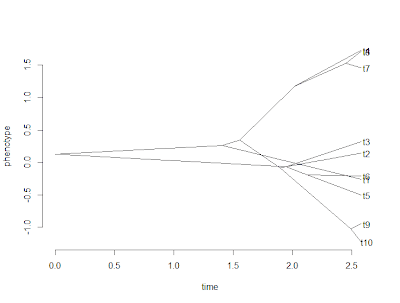> phenogram(tree,x,axes=list(trait=c(-2,2))) # change vertical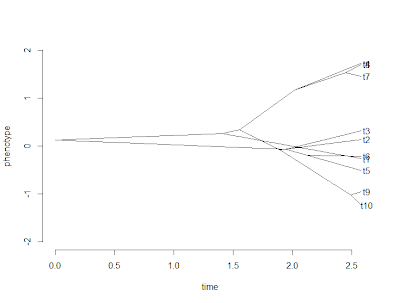> # change horizontal
> phenogram(tree,x,axes=list(trait=c(-2,2), time=c(1.5,max(nodeHeights(tree)))))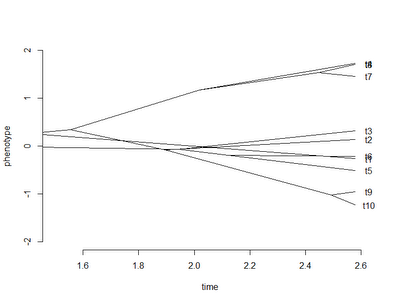It also works with a mapped discrete character. For instance:

> Q<-matrix(c(-2,2,2,-2),2,2)
> mtree<-sim.history(tree,Q)
> cols<-c("blue","red"); names(cols)<-c(1,2)
> phenogram(mtree,x,colors=cols,axes=list(trait=c(-2,2)))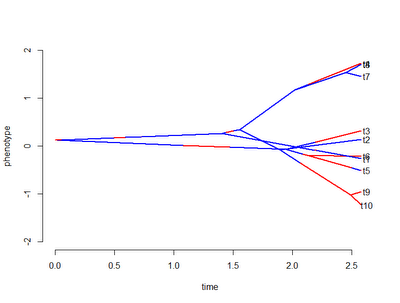Cool.

### New version of phylosig with control of multidimensional optimization migrated to the user

I just posted a new version of phylosig in which I have done two things to address the issue identified by Matt Johnson at Duke. He found that phylosig(...,method="lambda",se=se) failed to converge to the MLE of λ when the branches of the tree were rescaled to be much shorter in length (say to 1/1000 of their original length).

First, I changed the default starting values for ML optimization. In particular, for ML optimization of σ2 I had been using the REML estimate of σ2 conditioned on λ=1.0 and zero measurement error. (This is easy because this is just the mean square of the independent contrasts for the trait.) When we have lots of measurement error, this starting value puts us far past the MLE of σ2 for the full model. In addition, the log-likelihood surface is asymmetric around the MLE for σ2. This can be seen for the log-likelihood of the variance of a normal distribution via the following simple code:

> log.likelihood<-function(sig2,x,mu) sum(dnorm(x,mean=0,sd=sqrt(sig2),log=TRUE))
> x<-rnorm(n=100,sd=sqrt(2),mean=0)
> sig2<-1:100/10
> logL<-apply(as.matrix(sig2),1,log.likelihood,x=x,mu=0)
> plot(sig2,logL,"l")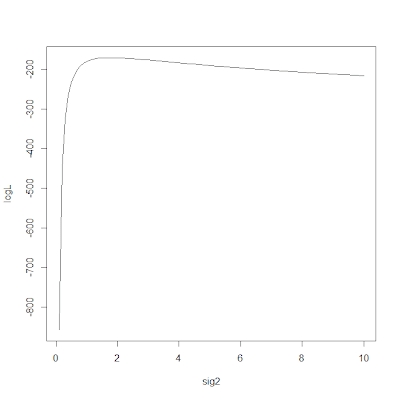Second, I have also migrated several aspects of the control of the internally used R base package multidimensional optimizer, optim, to the phytools user. In particular, the user can now control the control parameters of optim as well as the starting values for optimization. Users may also notice that I now return the value for convergence (zero is good) & the message on convergence returned by optim.

Direct link to this updated code is here. Note that this only affects optimization of λ with within-species sampling error, because this is the only situation in which optim is used.

Let's see how these new updates affect our ability to solve our tricky tree & dataset from before.

> res1000<-phylosig(tree1000,xe,method="lambda",se=se)
> res1000
\$lambda
 0.7298903
\$sig2
 1037.687
\$logL
 -360.4223
\$convergence
 0
\$message
 "CONVERGENCE: REL_REDUCTION_OF_F <= FACTR*EPSMCH"

OK, well, that's pretty good. Let's try an even more dramatically rescaled tree:

> tree100000<-tree
> tree100000\$edge.length<-tree\$edge.length/100000
> res100000<-phylosig(tree100000,xe,method="lambda",se=se)
> res100000
\$lambda
 0.6357282
\$sig2
 51171.73
\$logL
 -364.529
\$convergence
 0
\$message
 "CONVERGENCE: REL_REDUCTION_OF_F <= FACTR*EPSMCH"

OK, that's not terribly far off the MLE of λ (which is better than before) but it's still not right. In addition, the function thinks that it has converged. Now, let's try and take advantage of the control parameters for optim.

> res100000<-phylosig(tree100000,xe,method="lambda",se=se, control=list(factr=1))
> res100000
\$lambda
 0.7298907
\$sig2
 103768.9
\$logL
 -360.4223
\$convergence
 0
\$message
 "CONVERGENCE: REL_REDUCTION_OF_F <= FACTR*EPSMCH"

This is cool. Here, I decreased the factor over machine precision that is used to control convergence. Setting it to 1.0 means that optim will only accept that it has converged when (to the maximum numerical precision of R) it can now longer improve the likelihood. Why, then, should we not just fix control=list(factr=1)? (The default is 107.) Well, for most datasets this would just add extra computation time (possibly a lot).

All right - try and break this version! (Just don't try too hard please.)

### Issue in phylosig() revealed for very short trees

Matt Johnson, a graduate student at Duke, just identified an interesting problem with the phytools function phylosig. Basically, he was finding that phylosig(...,method="lambda",se=se) was giving discrepant results when he rescaled the branch lengths of the tree to be very short (say, scaling all edge lengths by 1/1000). This was a problem unique to the optimization of λ with sampling error in the estimation of species means. This immediately pointed towards the R base package multidimensional optimizer, optim, because no other methods in phylosig use optim aside from λ with sampling error.

To reproduce the error that he found, Matt suggested something along the lines of the following code:

> set.seed(2)
> require(phytools); require(geiger)
> tree<-pbtree(n=200,scale=1)
> x<-fastBM(lambdaTree(tree,0.7))
> se<-sqrt(rchisq(n=200,df=2)); names(se)<-tree\$tip.label
> e<-rnorm(n=200,sd=se)
> xe<-x+e
> res1<-phylosig(tree,xe,method="lambda",se=se)
> res1
\$lambda
 0.7298913
\$sig2
 1.037691
\$logL
 -360.4223

Ok, so far so good. Now let's try and rescale the tree to have really short branches. In theory this should not affect our estimate of phylogenetic signal.

> tree1000<-tree; tree1000\$edge.length<-tree\$edge.length/1000
> res1000<-phylosig(tree1000,xe,method="lambda",se=se)
> res1000
\$lambda
 0.9986187
\$sig2
 51435.36
\$logL
 -471.9413

Obviously, there's some kind of problem here. What I've been able to figure out is that it is a convergence issue - for some reason, convergence is more difficult for the larger value of σ2 that results from rescaling the branch lengths to have very short lengths. [Note that for any change to the branch lengths of the tree by factor 1/k, the fitted evolutionary rate should increase by factor k.] I've made some headway towards resolving this issue and will post more, along with an updated version of phylosig shortly.

## Sunday, February 5, 2012

### Printing a tree to file without branch lengths

A recent Google search string that led a user to the phytools blog was as follows:

"how to create newick tree without distances"

By this I presume they mean: how does one print a Newick tree to file (or screen) without branch lengths? In R, this is very straightforward.

Every phylo object stored in memory has three main items and a class attribute "phylo". The items are a m × 2 matrix edge (for m branches in the tree); a vector of length N containing all the tip names (tip.label); and an integer, Nnode, which indicates the number of internal nodes in the tree. Trees with branch lengths also have an m length vector edge.length containing the branch lengths for all the branches in the tree (in the same order as the rows in edge).

To print a tree without its branch lengths, it is simply necessary to set edge.length to NULL. That's it - piece of cake, right?

Let's try it.

> tr1<-rtree(10) # random tree
> write.tree(tr1) # write tree with edge lengths
 "(((t10:0.055,t6:0.599):0.3,(t9:0,((((t5:0.728,t1:0.624):0.142,t4:0.639):0.965,t3:0.396):0.562,(t7:0.383,t2:0.241):0.14):0.741):0.501):0.801,t8:0.138);"
> tr2<-tr1 # copy tree
> tr2\$edge.length<-NULL # get rid of edge lengths
> write.tree(tr2) # write tree without edge lengths
 "(((t10,t6),(t9,((((t5,t1),t4),t3),(t7,t2)))),t8);"

Of course, this works just as well with write.nexus (which writes a NEXUS format tree) or write.tree(...,file=filename) and write.nexus(...,file=filename).

## Saturday, February 4, 2012

### New phytools page

I have just moved all the content of my R phylogenetics (phytools) development page from its old Harvard server (here), which will soon start redirecting, to my faculty space at UMass Boston (here). I will keep the old URL active for as long as I can. In addition, I have made every attempt to update all the links of this blog to point to the new page. Hopefully, I haven't missed too many!

## Wednesday, February 1, 2012

### New version of brownie.lite accounting for sampling error

I just posted a new version of the phytools function brownie.lite which does the analysis of O'Meara et al. (2006), but also accounts for sampling error in the estimation of species means (basically following Ives et al. 2007).

Because of the gradual and haphazard way that I had been adding features to brownie.lite, the previous version of the function was become excessively messy & cumbersome. To remedy that problem, and to add this new feature to the function, I decided to totally re-program it. To see this, compare the new version here to its most recent predecessor (here). I did pull some code snippets from the original version and from other functions, such as evolvcv.lite.

The way it works is straightforward. In the case of, say, a single rate of evolution on all the branches of the tree, we optimize the following function for the log-likelihood (expressed in terms of the ancestral state at the root node, the evolution rate σ2, and the cophenetic matrix containing the heights above the root for the MRCAs of all the terminal species in the tree):

lik.single<-function(theta,y,C,se){
n<-length(y)
sig<-theta
a<-theta
E<-diag(se^2)
logL<-as.numeric(-t(y-a)%*%solve(sig*C+E)%*%(y-a)/2 -n*log(2*pi)/2 -determinant(sig*C+E)\$modulus/2)
return(-logL)
}

If we don't have sampling error, or we must choose to ignore it, no problem - we just feed the function a vector of zeroes and we are back to optimizing the model of O'Meara et al. & all prior versions of brownie.lite.

To see how this work, let's first simulate some data under the model:

> set.seed(1) # set seed for repeatability
> Q<-matrix(c(-1,1,1,-1),2,2) # model for discrete character
> tree<-sim.history(pbtree(n=300,scale=1),Q,anc=1) # simulate rate history
> x<-sim.rates(tree,c(1,10)) # simulate with two rates
> se<-rchisq(n=300,df=1) # species-specific sampling errors
> xe<-rnorm(n=300,mean=x,sd=se) # simulate means estimated with error
> names(xe)<-names(se)<-names(x)

Now, let's fit the O'Meara et al. model under three scenarios: first, let's use the true (in this case) known species means; second, let's use the "estimated" means (i.e., the means simulated with error) and fit the model, but fail to account for sampling error; and, finally, third, let's fit the O'Meara et al. model but accounting for sampling error in the estimation of species means. (In the output below I've excised some details for brevity.)

> model1<-brownie.lite(tree,x)
> model2<-brownie.lite(tree,xe)
> model3<-brownie.lite(tree,xe,se=se)
> model1
\$sig2.single
 4.198556
...
\$logL1
 -375.638
\$k1
 2
\$sig2.multiple
1          2
0.8732808 10.7110750
...
\$logL.multiple
 -287.0903
\$k2
 3
\$P.chisq
 2.08773e-40
...
> model2
\$sig2.single
 36.49996
...
\$logL1
 -700.0235
\$k1
 2
\$sig2.multiple
1        2
19.60967 64.56690
...
\$logL.multiple
 -673.3162
\$k2
 3
\$P.chisq
 2.700823e-13
...
> model3
\$sig2.single
 3.927711
...
\$logL1
 -516.407
\$k1
 2
\$sig2.multiple
1          2
0.9975472 10.5384272
...
\$logL.multiple
 -480.9778
\$k2
 3
\$P.chisq
 3.837931e-17
...

The most salient features of this analysis are, in my opinion, as follows: (1) the estimated rates for both model 1 (true species means) & model 3 (estimated means, but accounting for uncertainty) are quite close to the generating values; (2) the estimated rates for model 2 (estimated species means, not accounting for error) are too high, although the general trend (σ22>>σ12) is still evident; and (3) the "lost information" inherent in substituting estimated species means for their true values results in a decrease in power - that is, the log-likelihood difference between the one and two-rate models is much smaller (and the P-value larger) in models 2 & 3 (although in this case, the effect is so strong and the tree so large that all models are highly significant).

I have added this to a new, non-static version of phytools (v0.1-63) which can be downloaded here and installed from source.

Finally, I realized in updating this function that the calculation of the P-value for brownie.lite(...,test="simulation") is wrong for previous versions of brownie.lite, so anyone using that option should definitely update phytools!